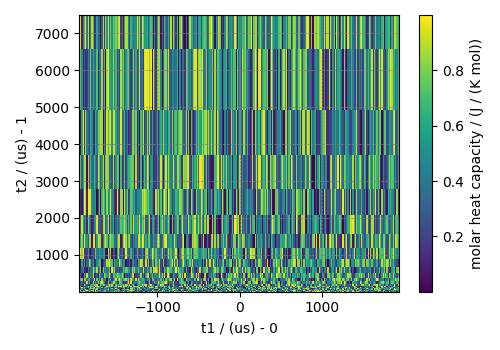# 2D{1} dataset with linear and monotonic dimensions¶

In the following example, we illustrate how one can covert a Numpy array into a CSDM object. Start by importing the Numpy and csdmpy libraries.

```import matplotlib.pyplot as plt
import numpy as np

import csdmpy as cp
```

Let’s generate a 2D NumPy array of random numbers as our dataset.

```data = np.random.rand(8192).reshape(32, 256)
```

Create the DependentVariable object from the numpy object.

```dv = cp.as_dependent_variable(data, unit="J/(mol K)")
```

Create the two Dimension objects.

```d0 = cp.LinearDimension(
count=256, increment="15.23 µs", coordinates_offset="-1.95 ms", label="t1"
)
```

Here, `d0` is a LinearDimension with 256 points and 15.23 µs increment. You may similarly set the second dimension as a LinearDimension, however, in this example, let’s set it as a MonotonicDimension.

```array = 10 ** (np.arange(32) / 8)
d1 = cp.as_dimension(array, unit="µs", label="t2")
```

The variable `array` is a NumPy array that is uniformly sampled on a log scale. To convert this array into a Dimension object, we use the `as_dimension()` method.

Creating the CSDM object.

```csdm_object = cp.CSDM(dependent_variables=[dv], dimensions=[d0, d1])
print(csdm_object.dimensions)
```

Out:

```[LinearDimension(count=256, increment=15.23 µs, coordinates_offset=-1.95 ms, quantity_name=time, label=t1, reciprocal={'quantity_name': 'frequency'}),
MonotonicDimension(coordinates=[1.00000000e+00 1.33352143e+00 1.77827941e+00 2.37137371e+00
3.16227766e+00 4.21696503e+00 5.62341325e+00 7.49894209e+00
1.00000000e+01 1.33352143e+01 1.77827941e+01 2.37137371e+01
3.16227766e+01 4.21696503e+01 5.62341325e+01 7.49894209e+01
1.00000000e+02 1.33352143e+02 1.77827941e+02 2.37137371e+02
3.16227766e+02 4.21696503e+02 5.62341325e+02 7.49894209e+02
1.00000000e+03 1.33352143e+03 1.77827941e+03 2.37137371e+03
3.16227766e+03 4.21696503e+03 5.62341325e+03 7.49894209e+03] us, quantity_name=time, label=t2, reciprocal={'quantity_name': 'frequency'})]
```

Plot of the dataset.

```plt.figure(figsize=(5, 3.5))
cp.plot(csdm_object)
plt.tight_layout()
plt.show()
```To serialize the file, use the save method.

```csdm_object.save("2D_1_dataset.csdf")
```

Total running time of the script: ( 0 minutes 0.181 seconds)

Gallery generated by Sphinx-Gallery